# Problem: Part AWhich of the following is the SI unit of angular momentum?a) N • m/sb) kg • m/sc) kg • m2/s2d) kg • m2/sPart BAn object has rotational inertia . The object, initially at rest, begins to rotate with a constant angular acceleration of magnitude . What is the magnitude of the angular momentum  of the object after time ?Express your answer in terms of, , and. =Part CA rigid, uniform bar with mass  and length rotates aboutthe axis passing through the midpoint of the bar perpendicular tothe bar. The linear speed of the end points of the bar is. What is themagnitude of the angular momentum  ofthe bar?Express your answer in terms of, , , andappropriate constants. =

###### FREE Expert Solution

Angular momentum:

$\overline{)\stackrel{\mathbf{⇀}}{\mathbf{L}}{\mathbf{=}}\stackrel{\mathbf{⇀}}{\mathbf{r}}{\mathbf{×}}\stackrel{\mathbf{⇀}}{\mathbf{p}}}$

$\overline{)\begin{array}{rcl}\stackrel{\mathbf{⇀}}{\mathbf{L}}& {\mathbf{=}}& \stackrel{\mathbf{⇀}}{\mathbf{I}}\mathbf{×}\stackrel{\mathbf{⇀}}{\mathbf{\omega }}\end{array}}$

Moment of inertia:

$\overline{){\mathbf{I}}{\mathbf{=}}{{\mathbf{\int }}}_{{\mathbf{0}}}^{{\mathbf{m}}}{{\mathbf{r}}}^{{\mathbf{2}}}{\mathbf{d}}{\mathbf{m}}}$

Part A

From the first equation of angular momentum:

p is in kg•m/s and r is in m

Substituting:

81% (232 ratings)###### Problem Details

Part A

Which of the following is the SI unit of angular momentum?

a) N • m/s

b) kg • m/s

c) kg • m2/s2

d) kg • m2/s

Part B

An object has rotational inertia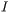. The object, initially at rest, begins to rotate with a constant angular acceleration of magnitude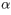. What is the magnitude of the angular momentum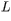of the object after time?

Express your answer in terms of, and.=

Part C

A rigid, uniform bar with mass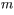and length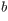rotates aboutthe axis passing through the midpoint of the bar perpendicular tothe bar. The linear speed of the end points of the bar is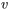. What is themagnitude of the angular momentumofthe bar?

Express your answer in terms of,,, andappropriate constants.=